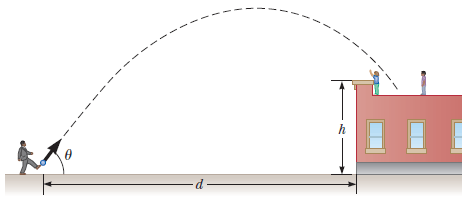# Problem: A playground is on the flat roof of a city school, 5 m above the street below (see figure). The vertical wall of the building is h = 6.50 m high, forming a 1.5-m-high railing around the playground. A ball has fallen to the street below, and a passerby returns it by launching it at an angle of θ = 53.0°; above the horizontal at a point d= 24.0 m from the base of the building wall. The ball takes 2.20 s to reach a point vertically above the wall.a) Find the speed at which the ball was launched. (Give your answer to two decimal places to reduce rounding errors in later parts.)b) Find the vertical distance by which the ball clears the wall.c) Find the horizontal distance from the wall to the point on the roof where the ball lands.

###### FREE Expert Solution

a)

Using the horizontal distance:

Δx = vx•t = (v0cosθ)t

v0 = Δx/(cosθ)t

v0 = 24.0/[cos (53.0°)(2.20)]###### Problem Details

A playground is on the flat roof of a city school, 5 m above the street below (see figure). The vertical wall of the building is h = 6.50 m high, forming a 1.5-m-high railing around the playground. A ball has fallen to the street below, and a passerby returns it by launching it at an angle of θ = 53.0°; above the horizontal at a point d= 24.0 m from the base of the building wall. The ball takes 2.20 s to reach a point vertically above the wall.a) Find the speed at which the ball was launched. (Give your answer to two decimal places to reduce rounding errors in later parts.)

b) Find the vertical distance by which the ball clears the wall.

c) Find the horizontal distance from the wall to the point on the roof where the ball lands.